# Chemical Reactions Chemical Reactions Describing Chemical Reactions Indications

• Slides: 29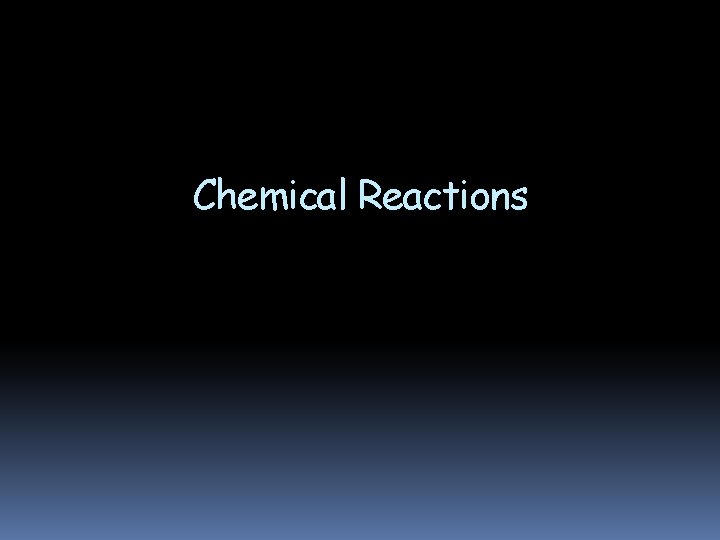Chemical ReactionsChemical Reactions Describing Chemical Reactions Indications of a Chemical Reaction Characteristics of Chemical Equations Balancing Chemical Equations Types of Reactions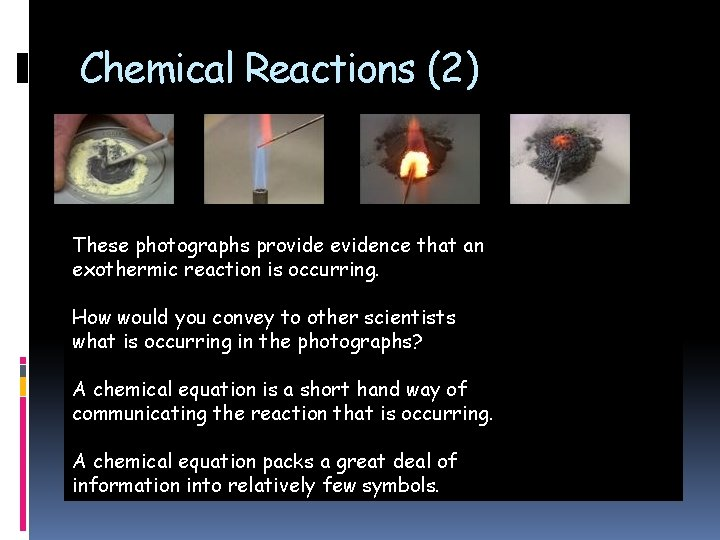Chemical Reactions (2) These photographs provide evidence that an exothermic reaction is occurring. How would you convey to other scientists what is occurring in the photographs? A chemical equation is a short hand way of communicating the reaction that is occurring. A chemical equation packs a great deal of information into relatively few symbols.Chemical Reactions (3) A chemical reaction is the process by which one or more substances (reactants) are changed into one or more different substances (products). According to the law of conservation of mass, the total mass of reactants must equal the total mass of products for any given chemical reaction.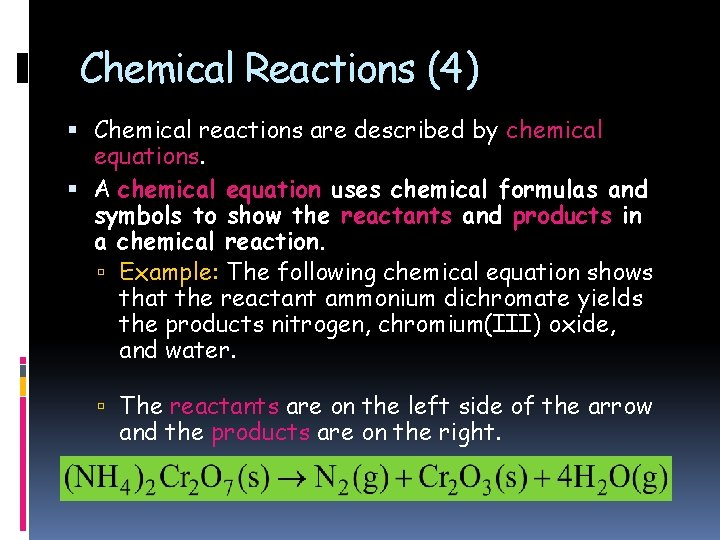Chemical Reactions (4) Chemical reactions are described by chemical equations. A chemical equation uses chemical formulas and symbols to show the reactants and products in a chemical reaction. Example: The following chemical equation shows that the reactant ammonium dichromate yields the products nitrogen, chromium(III) oxide, and water. The reactants are on the left side of the arrow and the products are on the right.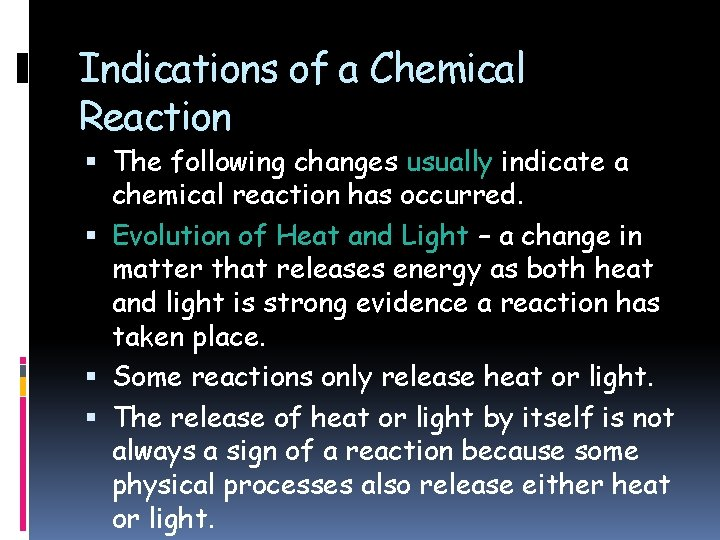Indications of a Chemical Reaction The following changes usually indicate a chemical reaction has occurred. Evolution of Heat and Light – a change in matter that releases energy as both heat and light is strong evidence a reaction has taken place. Some reactions only release heat or light. The release of heat or light by itself is not always a sign of a reaction because some physical processes also release either heat or light.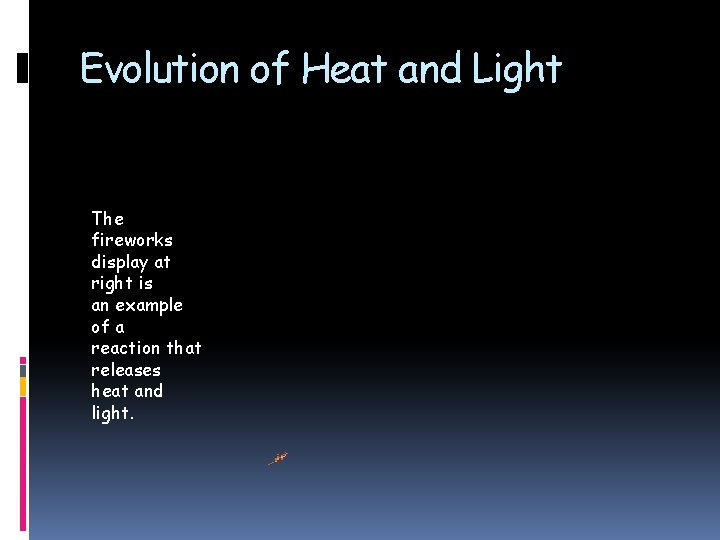Evolution of Heat and Light The fireworks display at right is an example of a reaction that releases heat and light.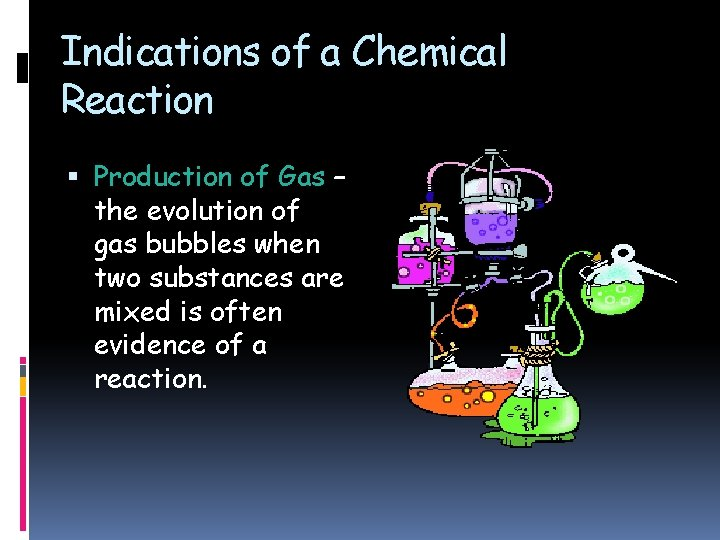Indications of a Chemical Reaction Production of Gas – the evolution of gas bubbles when two substances are mixed is often evidence of a reaction.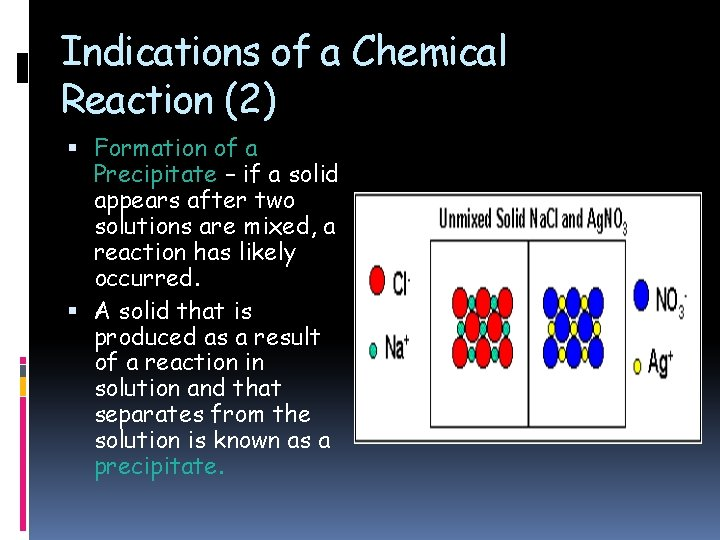Indications of a Chemical Reaction (2) Formation of a Precipitate – if a solid appears after two solutions are mixed, a reaction has likely occurred. A solid that is produced as a result of a reaction in solution and that separates from the solution is known as a precipitate.Indications of a Chemical Reaction (3) Color Change – a change in color is often an indication of a reaction.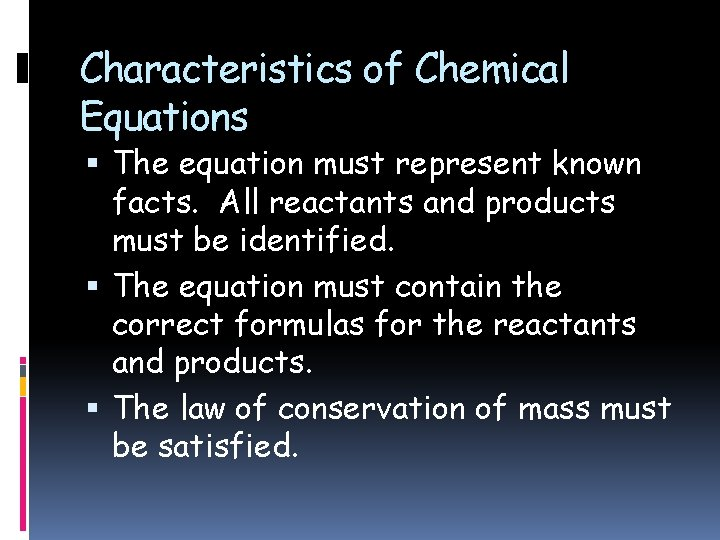Characteristics of Chemical Equations The equation must represent known facts. All reactants and products must be identified. The equation must contain the correct formulas for the reactants and products. The law of conservation of mass must be satisfied.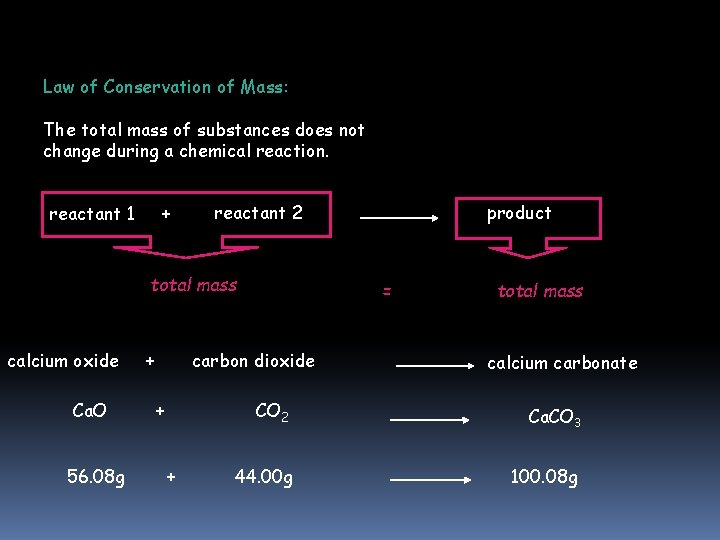Law of Conservation of Mass: The total mass of substances does not change during a chemical reaction. reactant 1 + reactant 2 total mass calcium oxide Ca. O 56. 08 g + = carbon dioxide + + product total mass calcium carbonate CO 2 Ca. CO 3 44. 00 g 100. 08 g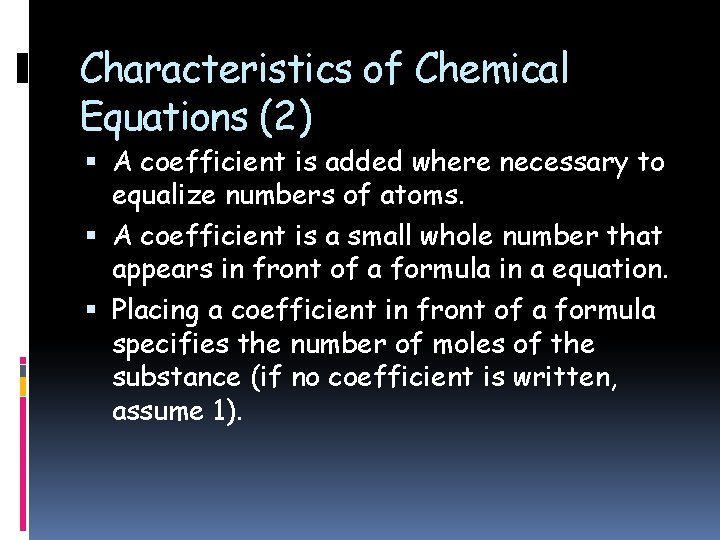Characteristics of Chemical Equations (2) A coefficient is added where necessary to equalize numbers of atoms. A coefficient is a small whole number that appears in front of a formula in a equation. Placing a coefficient in front of a formula specifies the number of moles of the substance (if no coefficient is written, assume 1).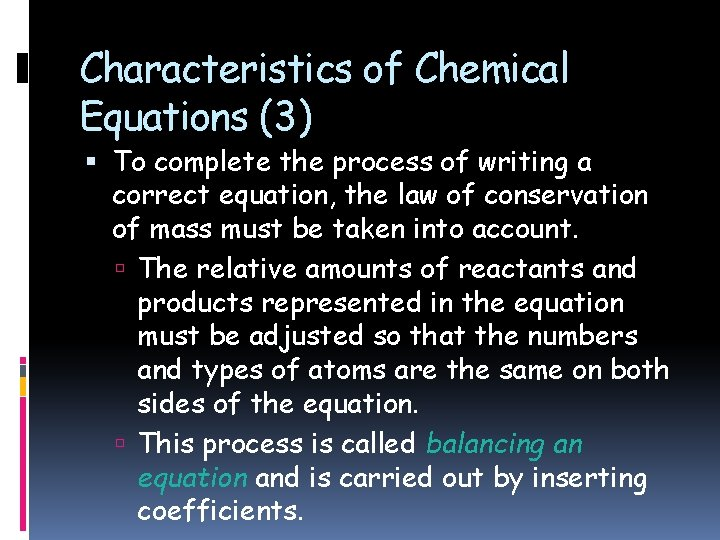Characteristics of Chemical Equations (3) To complete the process of writing a correct equation, the law of conservation of mass must be taken into account. The relative amounts of reactants and products represented in the equation must be adjusted so that the numbers and types of atoms are the same on both sides of the equation. This process is called balancing an equation and is carried out by inserting coefficients.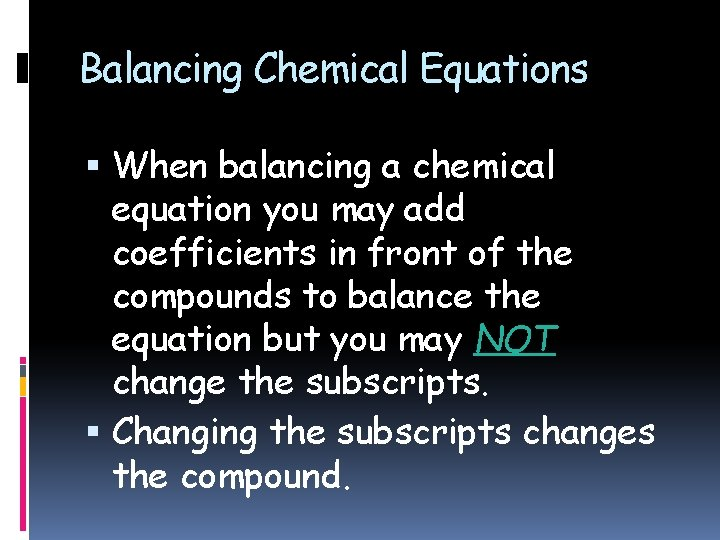Balancing Chemical Equations When balancing a chemical equation you may add coefficients in front of the compounds to balance the equation but you may NOT change the subscripts. Changing the subscripts changes the compound.How to Balance a Chemical Equation Mg + O 2 → Mg. O 1. The same number of each kind of atom must appear on both sides. So far, there is one atom of magnesium on each side of the equation. 2. But there are two oxygen atoms on the left and only one on the right. To balance the number of oxygen atoms, you need to double the amount of magnesium oxide by adding a coefficient of 2 (you cannot change the chemical formulas so the subscripts cannot be changed). Mg + O 2 → 2 Mg. OMg + O 2 → 2 Mg. O 3. This equation gives you two magnesium atoms on the right and only one on the left. So you need to double the amount of magnesium on the left by adding a coefficient of 2. 2 Mg + O 2 → 2 Mg. O 4. Now the equation is balanced. It has an equal number of each type of atom on both sides.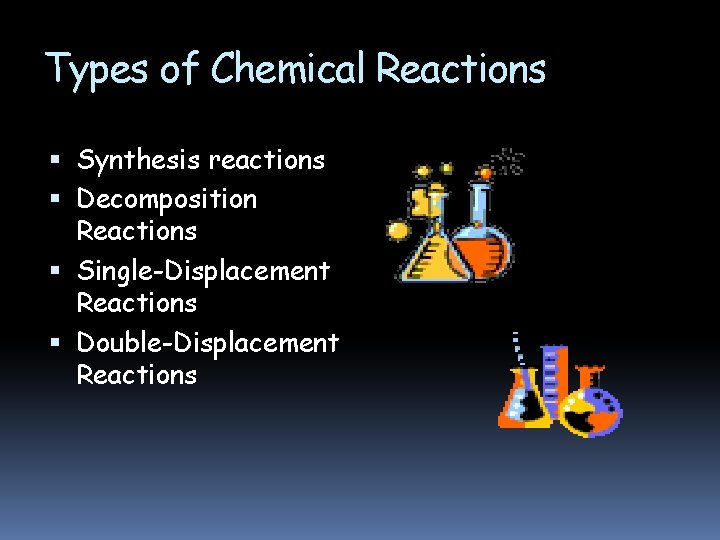Types of Chemical Reactions Synthesis reactions Decomposition Reactions Single-Displacement Reactions Double-Displacement ReactionsTypes of Chemical Reactions (2) So many chemical reactions can occur or are occurring that it would be impossible to predict their products if it was not possible to place many of them into categories. Synthesis reactions are one class of reactions in which substances combine to form a new compound.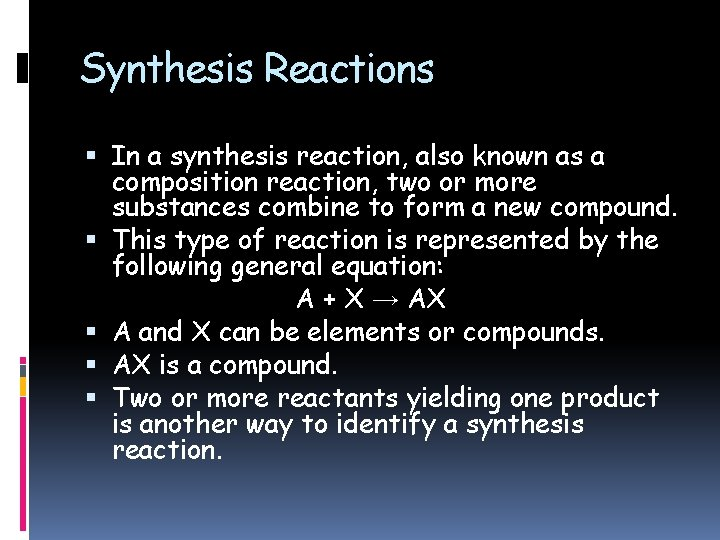Synthesis Reactions In a synthesis reaction, also known as a composition reaction, two or more substances combine to form a new compound. This type of reaction is represented by the following general equation: A + X → AX A and X can be elements or compounds. AX is a compound. Two or more reactants yielding one product is another way to identify a synthesis reaction.Synthesis Reactions (2) To visualize a synthesis reaction, look at the cartoon above. A skinny bird (reactant) and the worm (reactant) combine to make one product, the fat bird.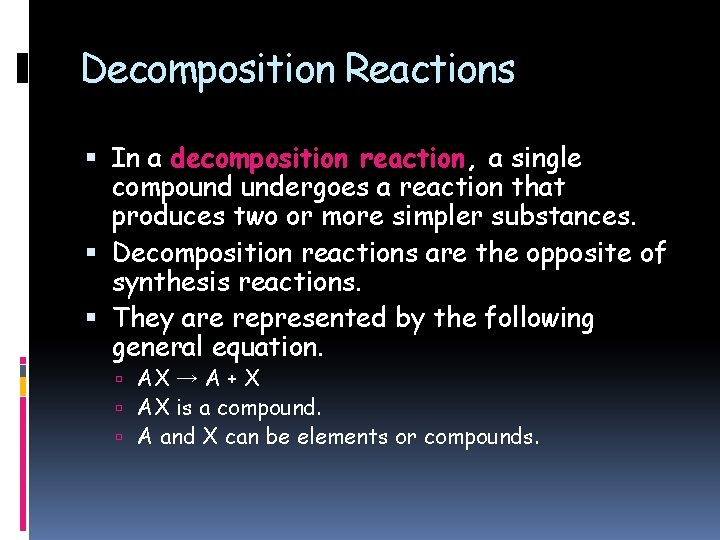Decomposition Reactions In a decomposition reaction, a single compound undergoes a reaction that produces two or more simpler substances. Decomposition reactions are the opposite of synthesis reactions. They are represented by the following general equation. AX → A + X AX is a compound. A and X can be elements or compounds.Decomposition Reactions (2) To visualize a decomposition reaction, look at the cartoon above. In this cartoon, the egg (reactant) which contained the turtle at one time, now has opened and the turtle (product) and egg shell (product) are now two separate substances.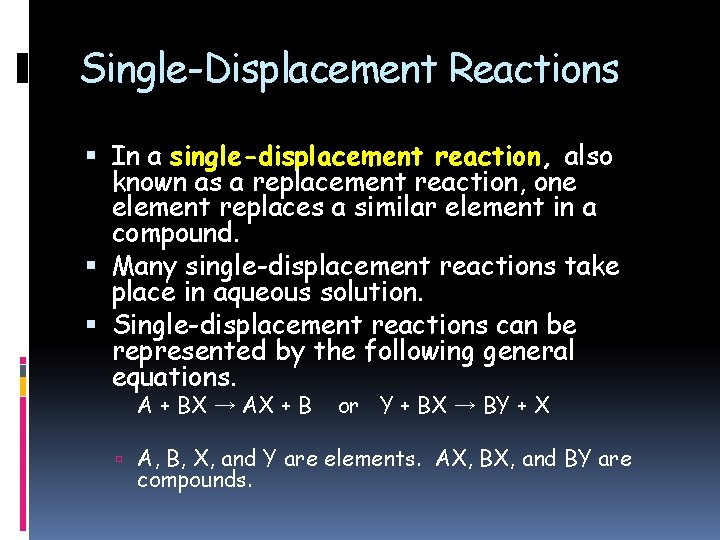Single-Displacement Reactions In a single-displacement reaction, also known as a replacement reaction, one element replaces a similar element in a compound. Many single-displacement reactions take place in aqueous solution. Single-displacement reactions can be represented by the following general equations. A + BX → AX + B or Y + BX → BY + X A, B, X, and Y are elements. AX, BX, and BY are compounds.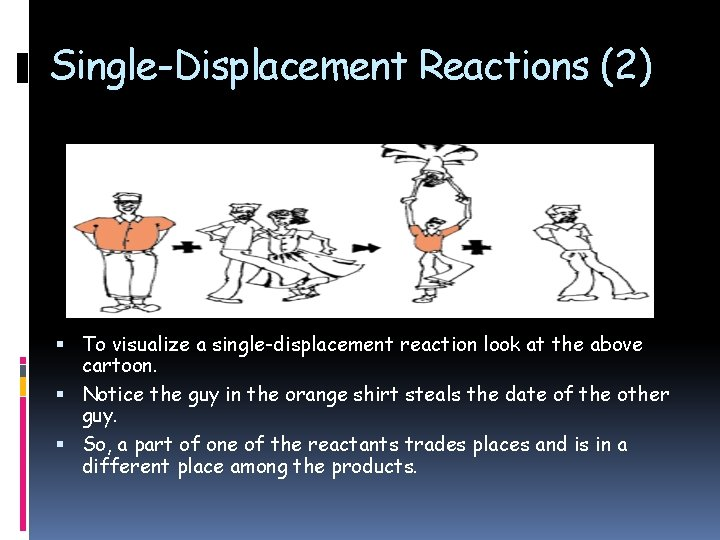Single-Displacement Reactions (2) To visualize a single-displacement reaction look at the above cartoon. Notice the guy in the orange shirt steals the date of the other guy. So, a part of one of the reactants trades places and is in a different place among the products.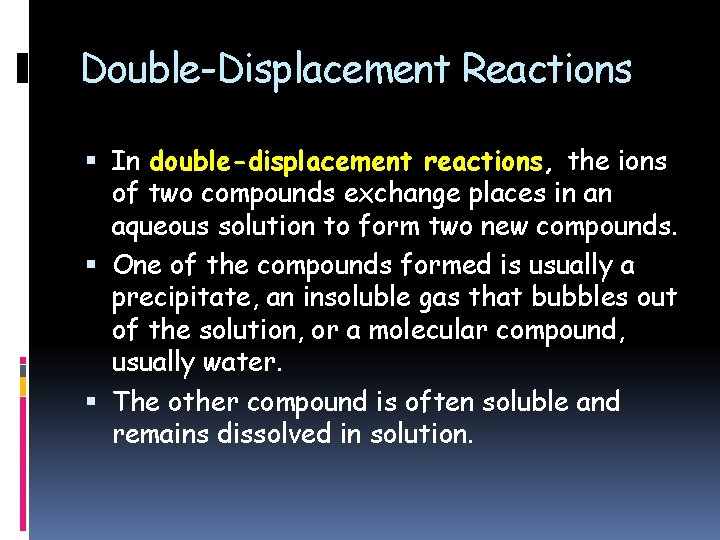Double-Displacement Reactions In double-displacement reactions, the ions of two compounds exchange places in an aqueous solution to form two new compounds. One of the compounds formed is usually a precipitate, an insoluble gas that bubbles out of the solution, or a molecular compound, usually water. The other compound is often soluble and remains dissolved in solution.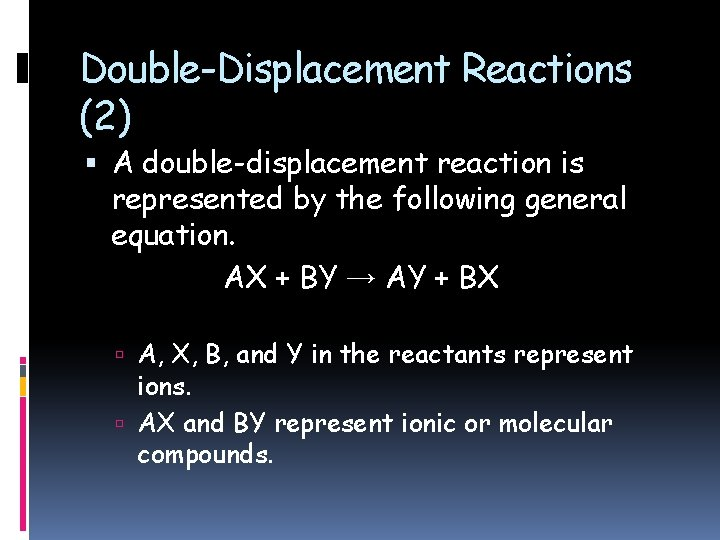Double-Displacement Reactions (2) A double-displacement reaction is represented by the following general equation. AX + BY → AY + BX A, X, B, and Y in the reactants represent ions. AX and BY represent ionic or molecular compounds.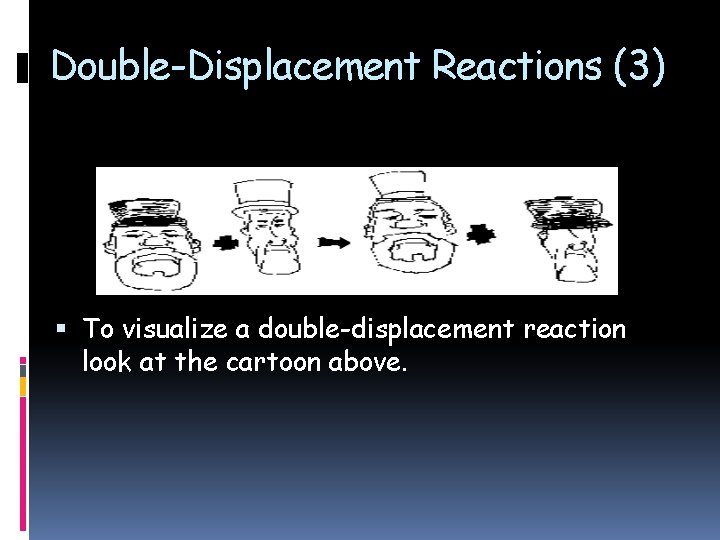Double-Displacement Reactions (3) To visualize a double-displacement reaction look at the cartoon above.